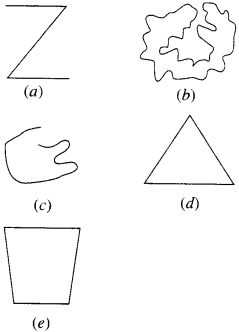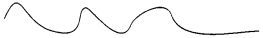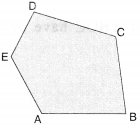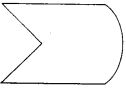NCERT Solutions for Class 6 Maths Chapter 4 Basic Geometrical Ideas Ex 4.2 are part of NCERT Solutions for Class 6 Maths. Here we have given NCERT Solutions for Class 6 Maths Chapter 4 Basic Geometrical Ideas Ex 4.2.

 Board CBSE Textbook NCERT Class Class 6 Subject Maths Chapter Chapter 4 Chapter Name Basic Geometrical Ideas Exercise Ex 4.2 Number of Questions Solved 5 Category NCERT Solutions

## NCERT Solutions for Class 6 Maths Chapter 4 Basic Geometrical Ideas Ex 4.2

Question 1.
Classify the following curves as
(i) open
(ii) closed.Solution :
(i) a, c
(ii) b,d,e.

Question 2.
Draw rough diagrams to illustrate the following :
(a) Open curve
(b) Closed curve.
Solution :
(a)(b)Question 3.
Draw any polygon and shade its interior.
Solution :Question 4.
Consider the given figure and answer the questions:
(a) Is it a curve?(b) Is it closed?
Solution :
(a) Yes! It is a curve
(b) Yes! It is closed.

Question 5.
Illustrate, if possible, each one of the following with a rough diagram :
(a) A closed curve that is not a polygon
(b) An open curve made up entirely of line segments.
(c) A polygon with two sides.
Solution :
(a)(b)(c) Not possible.

We hope the NCERT Solutions for Class 6 Maths Chapter 4 Basic Geometrical Ideas Ex 4.2 help you. If you have any query regarding NCERT Solutions for Class 6 Maths Chapter 4 Basic Geometrical Ideas Ex 4.2, drop a comment below and we will get back to you at the earliest.